0%

## 总结

• 这篇文章提出了一种新的解决detection的网络结构
• 不同于R-CNN系列的多阶段结构，YOLO只是用一个传统CNN结构就输出了所有的预测信息
• YOLO的优势：
• 快，每秒45张图片（平均每张图片耗时22ms）同时还有一个不错的mAP: 63.4
• 处理整张图片而不是特定的一个proposal，有更少的背景误分类
• 特征更泛化（通过更高的艺术品人像识别率来证明）
• YOLO的劣势：
• 每个框的bounding box数目受限
• 每个框至多属于一类
• 由于上述原因对于小物体的检测效果不好
• Detection原理
• 把图像分为 S * S块
• 每个块输出B个bounding box信息
• 每个bounding box信息包含五项
• bounding box的中心的x y坐标
• bounding box的长和宽
• 这个bounding box包含物体的信心分
• 共计五项
• 此外每个块还输出在包含物体的情况下，物体所属分类的概率
• 模型就是CNN网络，综上模型最后一层的输出是S S (B * 5 + num of classes)的一个tensor
• 训练细节
• 使用ImageNet pre-train + fine-tune
• 在fine-tune阶段，把input_size扩大一倍（detection任务需要更清晰的图片）
• 对x,y,h,w进行归一化
• 平衡大小bounding box对损失的影响，对h,w开平方处理
• 对包含物体的块的损失部分做加权处理(*5)
• 对不包含物体的块的损失部分做减小权重处理(*0.5)
• 具体公式见loss function部分

### VOC测试结果

• time: 22ms
• VOC 2007: 63.4%
• VOC 2012: 57.9%

## Abstract

We present YOLO, a new approach to object detection.

We frame object detection as a regression problem instead of classification problem.

less likely to predict false positives on background

## Introduction

YOLO’s structure is refreshingly simple: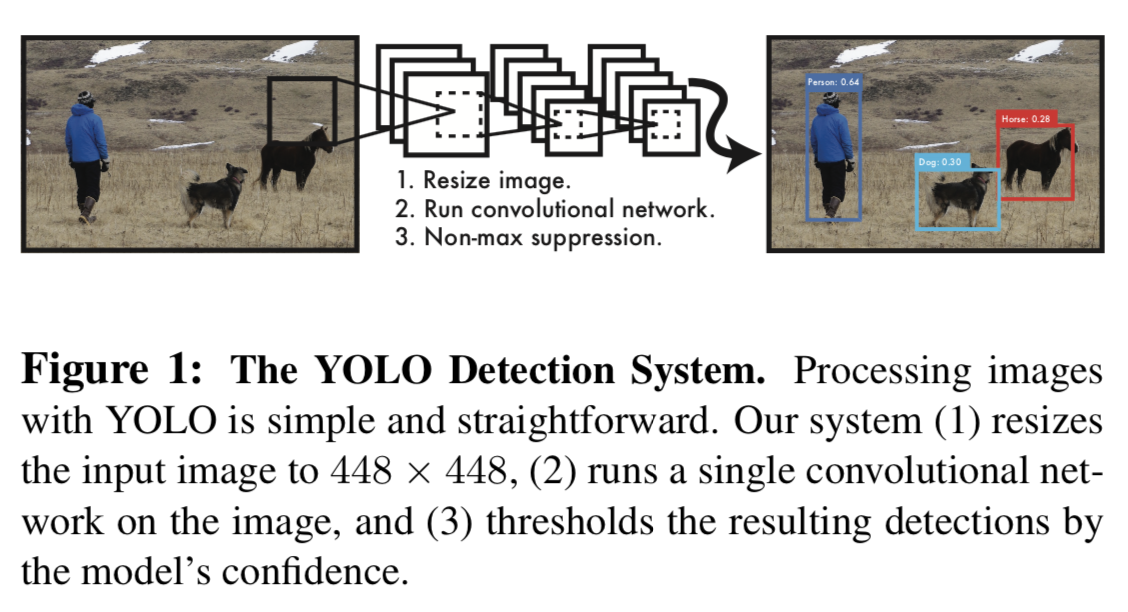This unified model has several benefits:

• extremely fast
• makes less background errors because of reasoning globally about image
• learns generalizable representations of objects

## Unified Detection

• Our system divides the input image into an S × S grid.
• If the center of an object falls into a grid cell, that grid cell is responsible for detecting that object.
• Each grid cell predicts B bounding boxes and confidence scores for those boxes.
• this confident scores reflect how confident the model is that the box contains an object
• and also how accurate it think the box is that it predicts
• We define confidence as $Pr(object) * IOU^{truth}_{pred}$:
• if no object exists in this cell, score should be zero
• otherwise we want the confident score to equal the intersection over union between the predict box and ground truth
• Each bounding consists of 5 predictions: x, y, w, h, confidence
• (x, y) represent the center of the box
• w and h represent wide and hight of the box
• Each grid cell also predicts C conditional class probabilities, $Pr(Class_i| Object)$
• We can get class-specific confidence scores for each box by:$Pr(Class_i| Object) * Pr(object) * IOU^{truth}_{pred} = Pr(class_i) * IOU^{truth}_{pred}$

In this paper we use S = 7, B = 2, C = 20
So our final prediction is a 7 7 30 tensor

### Network Design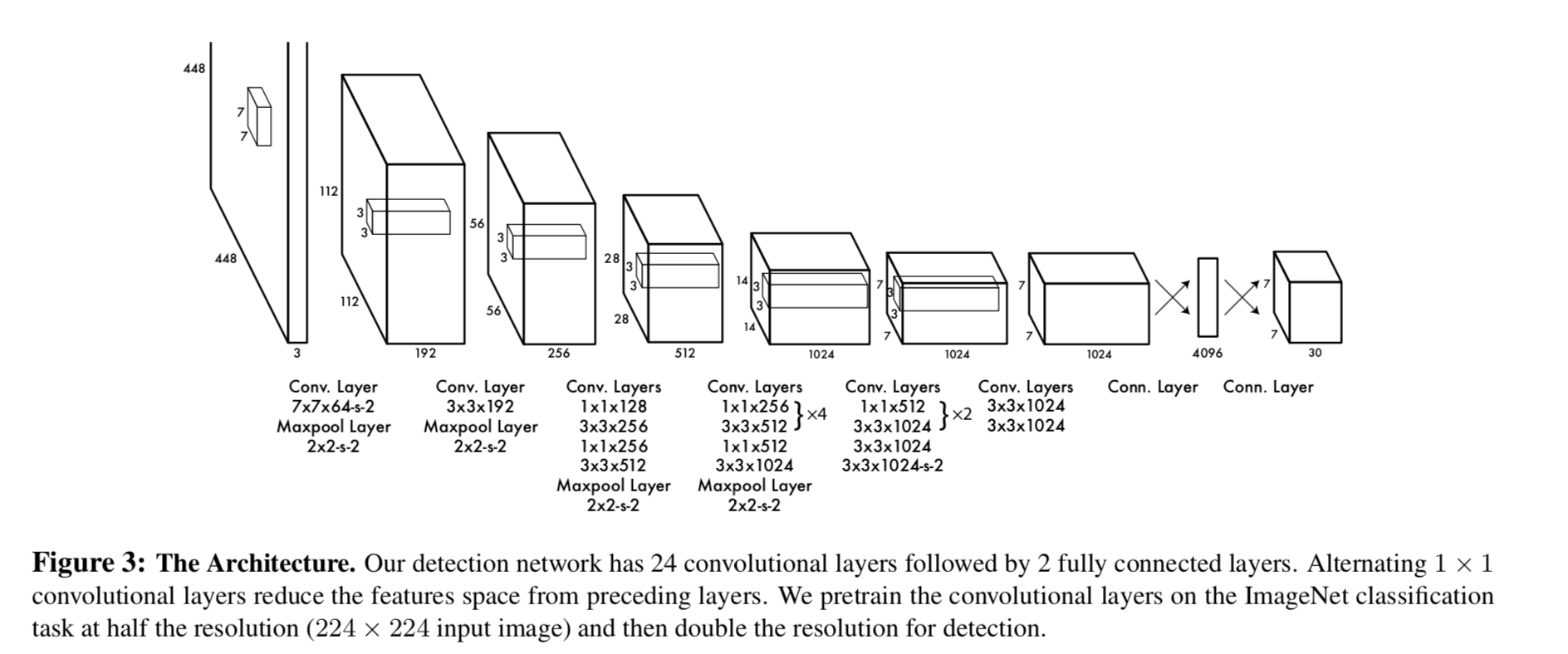Fast YOLO uses a neural network with fewer convolutional layers (9 instead of 24) and fewer filters in those layers

### Training

• pretrain convolutional layers on the ImageNet 1000-class competition dataset
• first 20 convolutional layers
• train for a week(amazing)
• convert the model to perform detection
• add four convolutional layers and two fully connected layers
• change input resolution from 224 224 to 448 448
• normalize the bounding box width and height by the image width and height so that they fall between 0 and 1(x, y too)
• use leaky rectified linear activation
• use sum-squared error
• easy to optimize
• weights localization error equally with classification error(flaw)
• the gradient from cells which have no object often overpowering the gradient from cells that do contain objects(flaw)
• equally weights errors in large boxes and small boxes(flaw)
• to remedy these flaws of sum-squared error
• increase the loss from bounding box coordinate predictions
• decrease the loss from confidence predictions for boxes that don’t contain objects
• set $\lambda_{coord} = 5$ and $\lambda_{noobj} = 0.5$ to accomplish this
• predict square root of the bounding box width and height instead of the width and height directly
• assign one predictor to be “responsible” for predicting an object based on which prediction has the highest current IOU with the ground truth

loss function:

• only penalizes classification error if an object is present in that grid cell
• only penalizes bounding box coordinate error if that predictor is “responsible” for the ground truth box

### Inference

• very fast
• use Non-maximal suppression to fix multiple detections

### Limitations of YOLO

• only predicts two boxes
• only have one class
• struggle to generalize to objects in new or unusual aspect ratios or configurations
• treats errors the same in small bounding boxes versus large bounding boxes

skip

## Experiments

skip

### VOC 2007 Error Analysis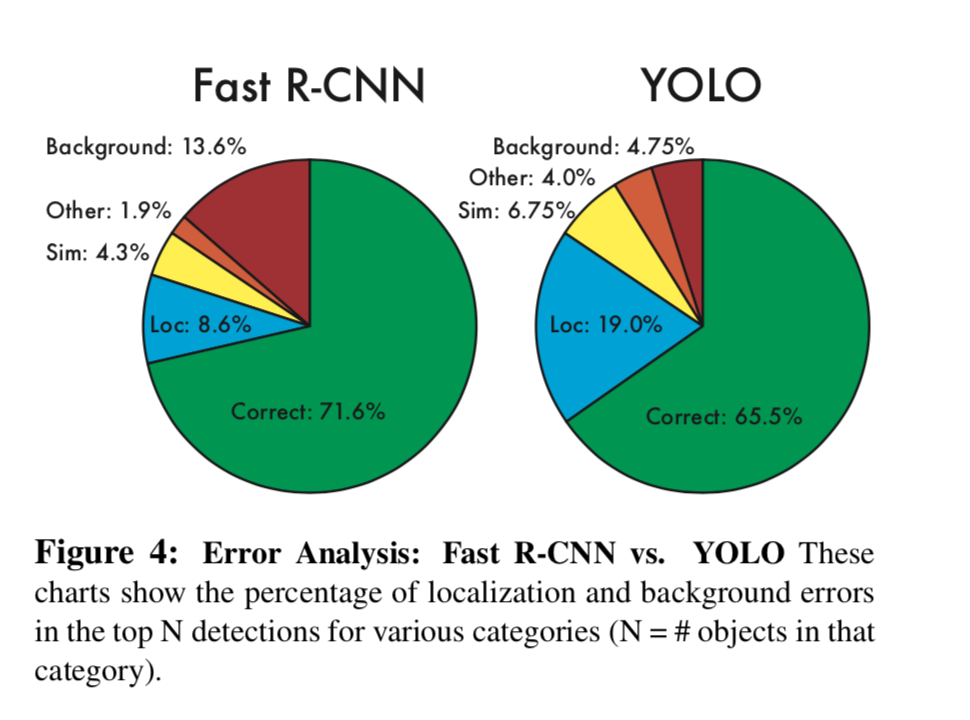• YOLO struggles to localize objects correctly
• Fast R-CNN

### Combining Fast R-CNN and YOLO

By using YOLO to eliminate background detections from Fast R-CNN we get a significant boost in performance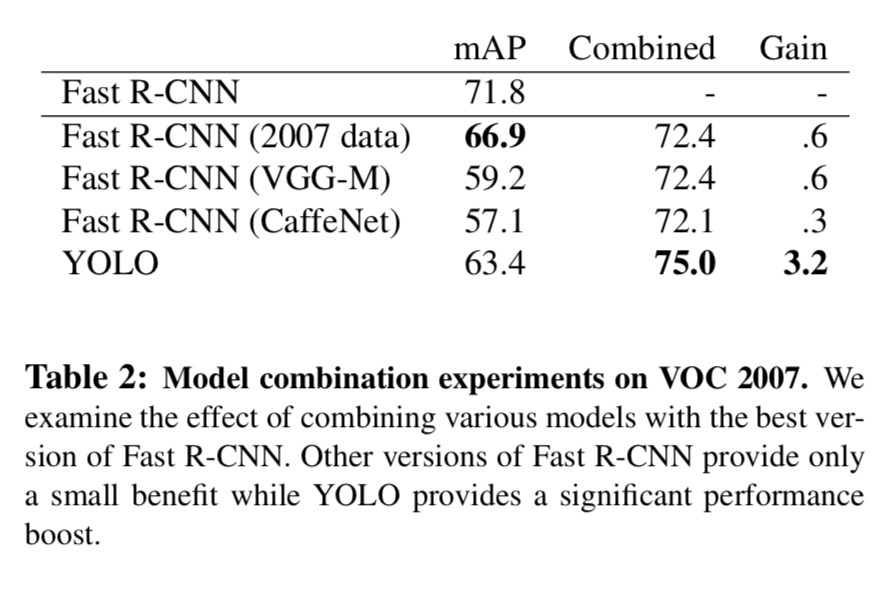### VOC 2012 Results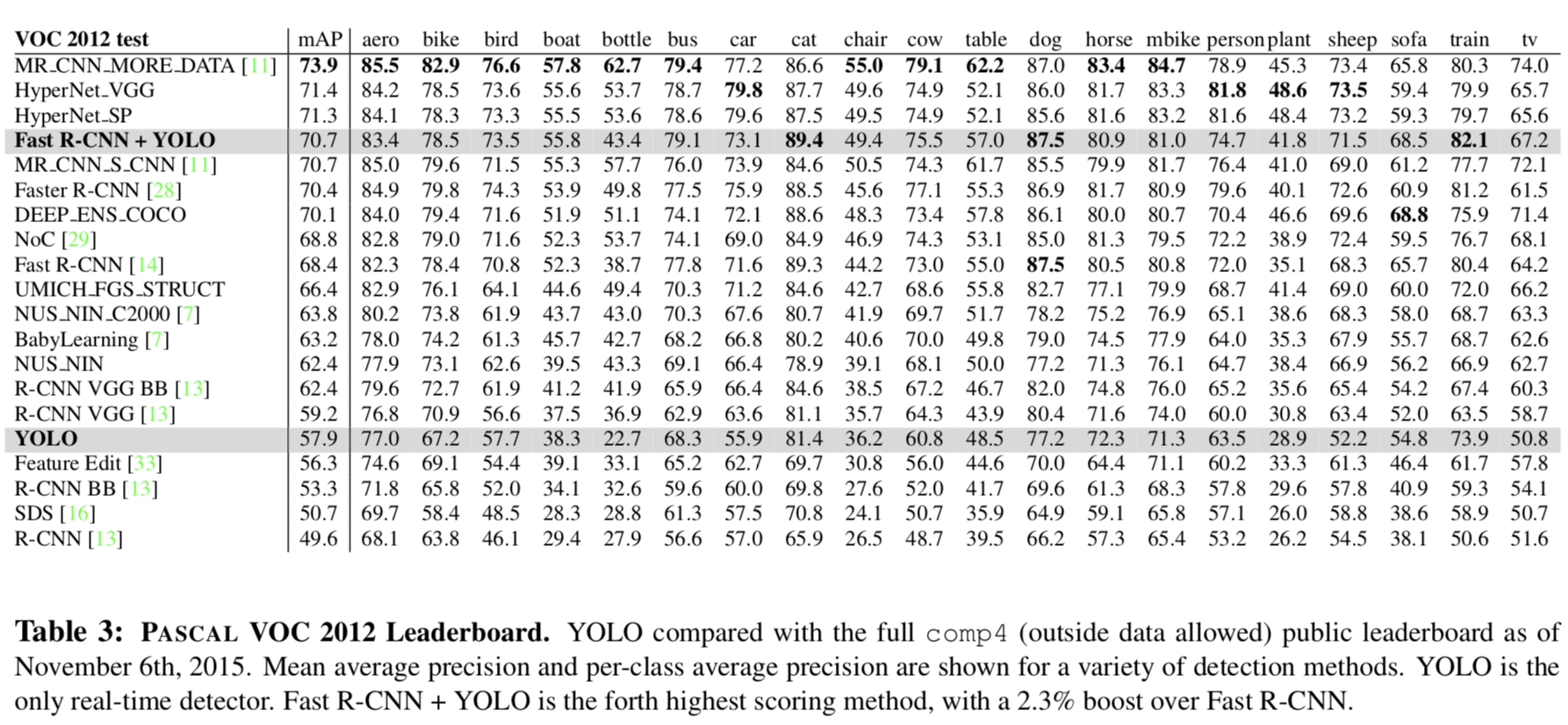### Generalizability: Person Detection in Artwork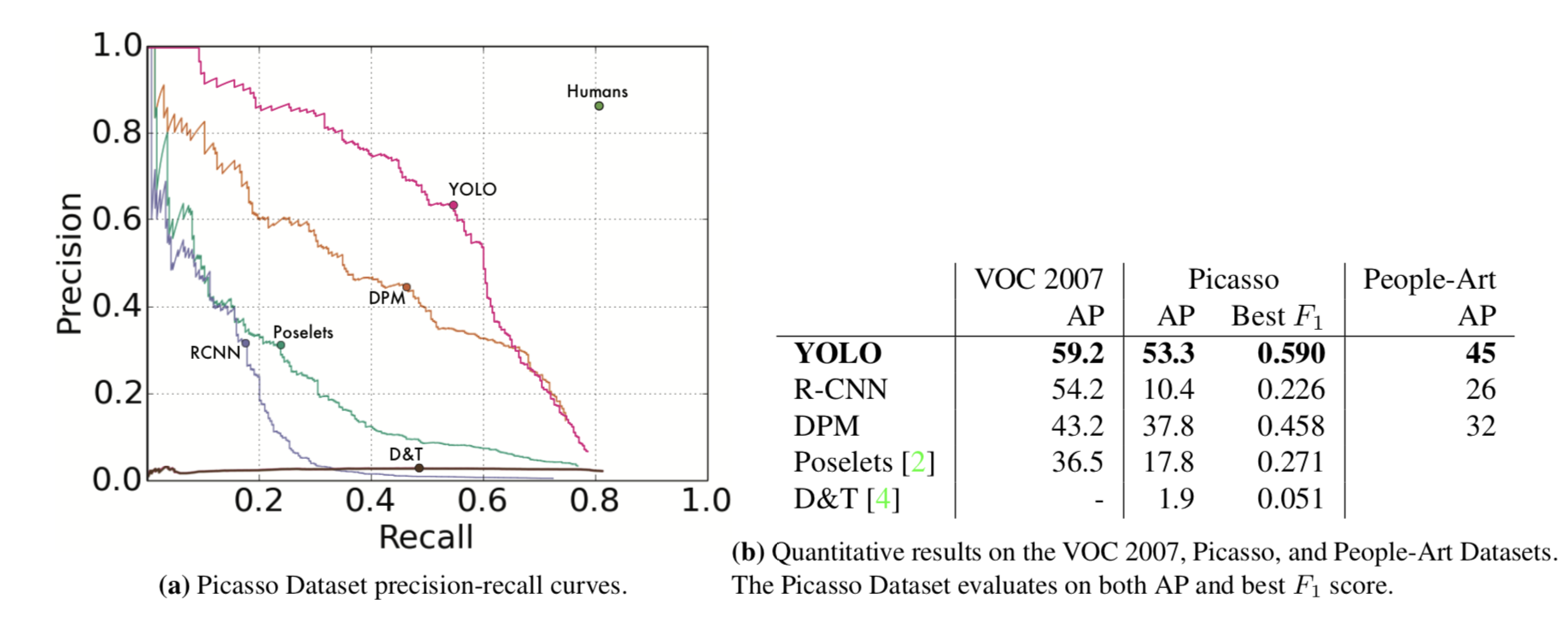YOLO models the size and shape of objects, as well as relationships between objects and where objects commonly appear

## Real-Time Detection In The Wild

perform well on webcam

skip